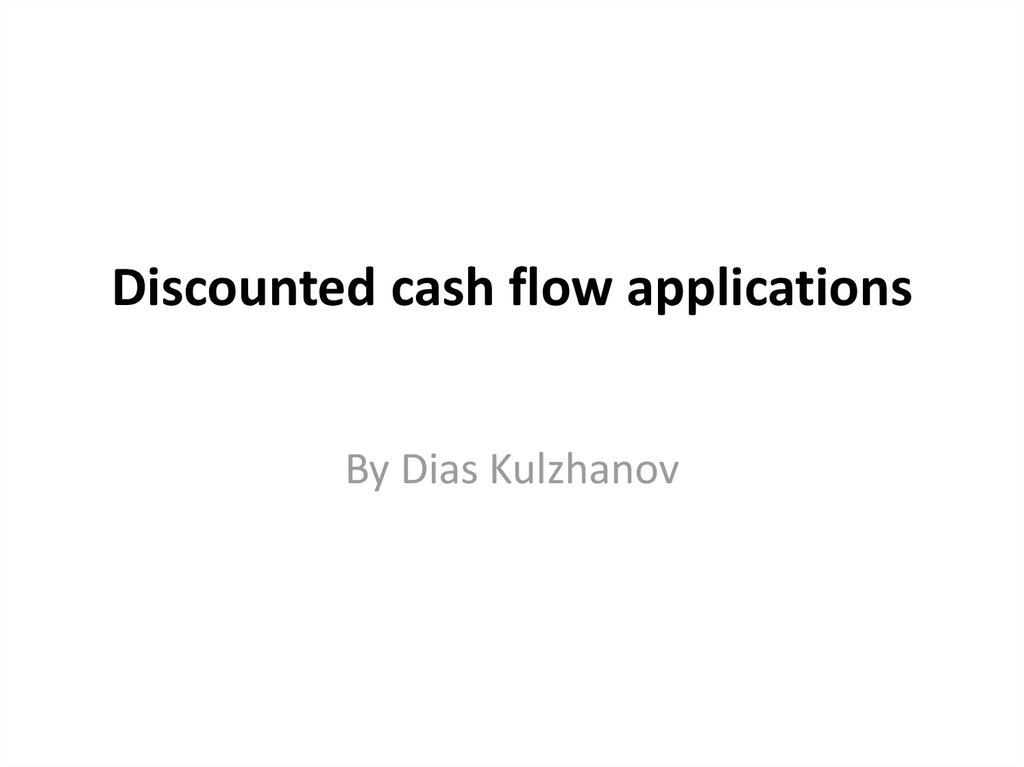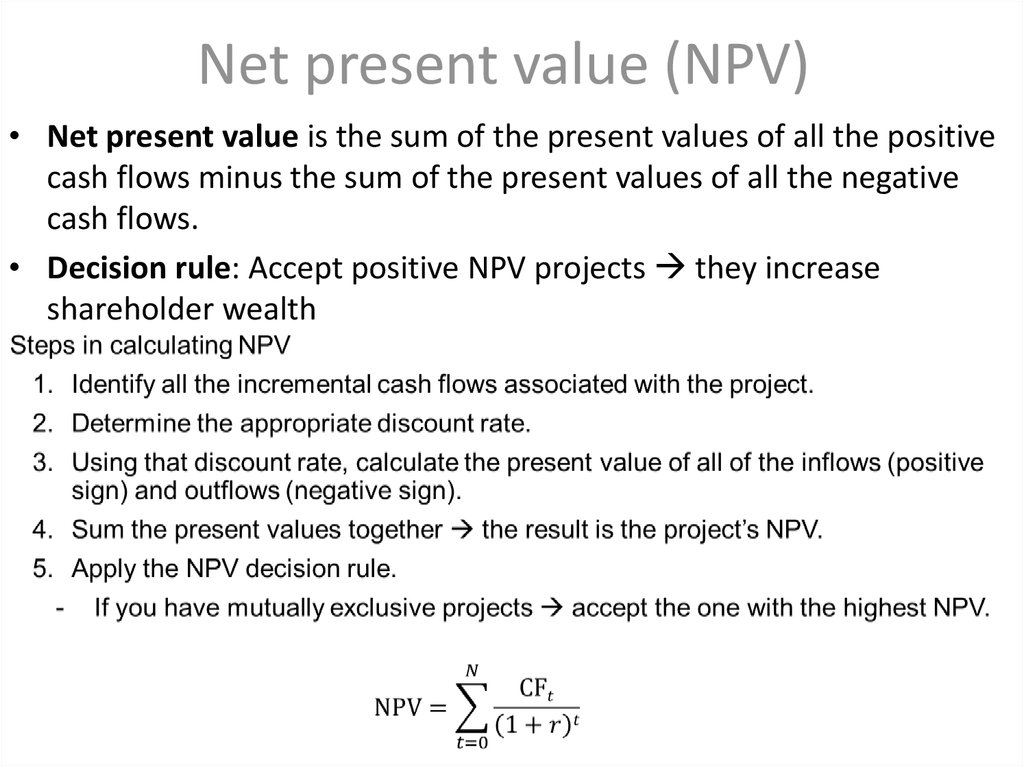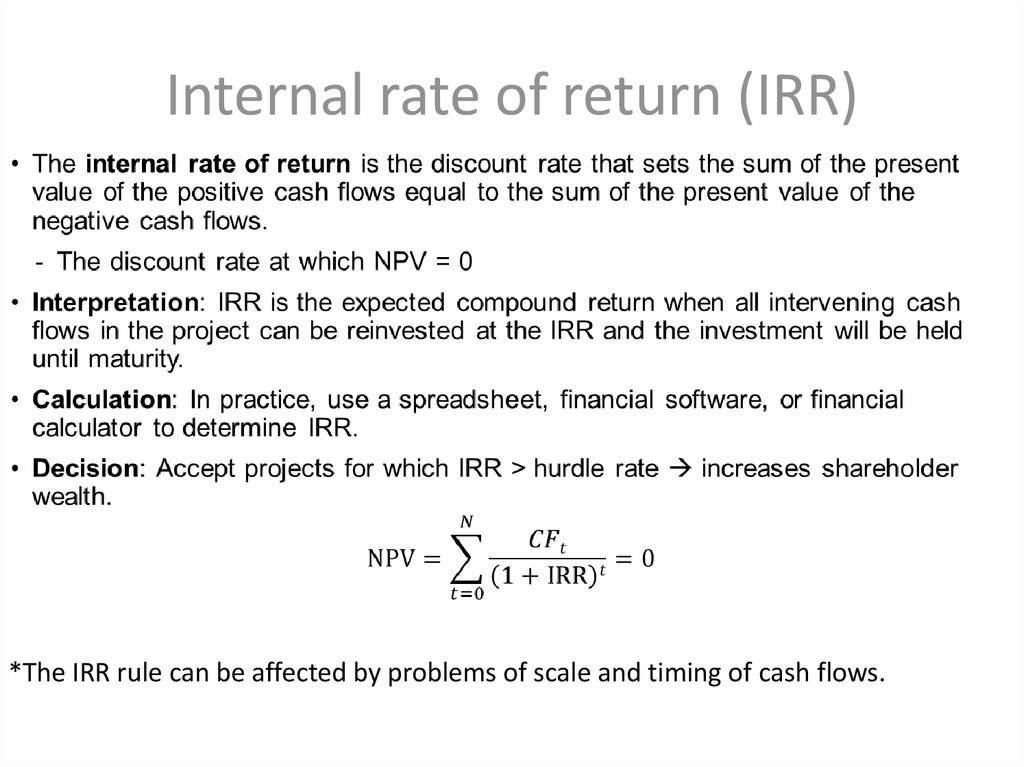# Discounted cash flow applications

## 1. Discounted cash flow applications

By Dias Kulzhanov

## 2. Net present value (NPV)

• Net present value is the sum of the present values of all the positive
cash flows minus the sum of the present values of all the negative
cash flows.
• Decision rule: Accept positive NPV projects they increase
shareholder wealth

## 3. Internal rate of return (IRR)

*The IRR rule can be affected by problems of scale and timing of cash flows.

## 4. NPV vs. IRR

• If projects are independent, the decision to invest in one does not
preclude investment in the other.
– NPV and IRR will yield the same investment decisions.
• Projects are mutually exclusive if the selection of one project
precludes the selection of another project project selection is
determined by rank.
– NPV and IRR may give different ranks when
• The projects have different scales (sizes)
• The timing of the cash flows differs
– If projects have different ranks use NPV

## 6. Money-weighted Rate of return

• The money-weighted rate of return is the internal rate of return on a
portfolio, taking account of all cash flows.
• Money-weighted rate of return can be appropriate if the investor
exercises control over additions and withdrawals to the portfolio.

## 7. Time-Weighted Rate of return

• Time-weighted rate of return is the standard in the investment
management industry.
• The time-weighted rate of return removes the effects of timing and
amount of withdrawals and additions to the portfolio and reflects the
compound rate of growth of one unit of currency invested over a
stated measurement period.

## 8. TWR vs. MWR

• Money-weighted returns place greater weight on those periods in
which investment is higher and, therefore, give a “better” picture of
the actual investor experience.
• Time-weighted returns remove the effect of inflows and outflows to
the portfolio and are, therefore, a better indicator of managerial skill.

## 9. Differing Money market Yields

• Instruments that mature in less than a year a known as money
market instruments
• There are a number of different conventions for calculating yields on
money market instruments.
– Bank discount yield
– Holding period yield
– Effective annual yield
– Money market yield

## 10. Bank discount yield

• By convention, Treasury instruments of less than a year in original
maturity (T-bills) have yield quoted on a bank discount basis.

## 11. Holding period yield (HPY)

*Treasury bill, HPY = D/P0

## 13. Money market yield

• This convention makes the quoted yield on a T-bill comparable to
yield quotations on interest-bearing money market instruments that
pay interest on a 360-day basis.
*For a Treasury bill, money market yield can be obtained from the bank
discount yield using rMM = (360 x rBD)/(360 − t xrBD).

## 14. Yield conversions

• We can convert back and forth using holding period yield, which is
common to all of the calculations.

## 15. Bond-equivalent yield (BEY)

• An annualized periodic yield wherein the process of annualizing is
accomplished by multiplying the periodic yield by the number of
periods in a year.
• By convention, the yield on most bonds is expressed as a bondequivalent yield instead of as an equivalent annual yield.
• Problem: This process ignores intraperiod compounding.
• Calculating a BEY:
– Compute the period yield to maturity (YTM).
– Multiply the periodic YTM by the number of periods in a year.
• For example: YTM x 2 for semiannual, YTM x 4 for quarterly